Custom SearchANGLE BETWEEN TWO LINES When two lines intersect, the angle between them is defined as the angle through which one of the lines must be rotated to make it coincide with the other line. For example, the angle(the Greek letter phi) in figure 1-7 is the acute angle between lines L, and L2. Referring to figure 1-7,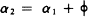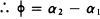We will determine the value of + directly from the slopes of lines L, and L2, as follows: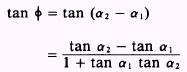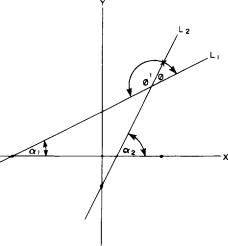Figure 1-7.-Angle between two lines. We obtain this result by using the trigonometric identity for the tangent of the difference between two angles. Trigonometric identities are discussed in chapter 6 of Mathematics, Volume 2-A, NAVEDTRA 10062. Recalling that the tangent of the angle of inclination is the slope of the line, we havesuch that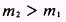Substituting these expressions in the tangent formula derived in the above discussion, we have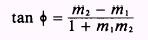EXAMPLE: Referring to figure 1-7, find the acute angle between the two lines that have m, =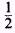and m2 = 2 for their slopes. SOLUTION:such thator, referring to Appendix 1,NOTE: To find the obtuse angle between lines L, and L2, just subtract the acute angle between L, and L2 from 180 . Referring to figure 1-7,If the obtuse angle in the previous example was to be found, thenIf one of the lines was parallel to the Y axis, its slope would be infinite. This would render the slope formula for tan + useless, because an infinite value in both the numerator and denominator of the fractionproduces an indeterminate form. 1 + m,m2 However, if only one of the lines is known to be parallel to the Y axis, the tangent of + may be expressed by another method. Suppose that L2 (fig 1-7) was parallel to the Y axis. Then we would have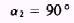If L, has a positive slope, then the acute angle between L, and LZ would be found byIf L, has a negative slope, then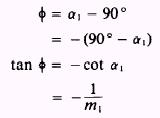As before, the obtuse angle can be found by usingPRACTICE PROBLEMS: 1. Find the acute angle between the two lines that have m, = 3 and m2 = 7 for their slopes. 2. Find the acute angle between two lines whose slopes are m, = 0 and m2 = 1. (m, = 0 signifies that line L, is horizontal and the formula still holds.) 1-12 3. Find the acute angle between the Y axis and a line with a slope of m = - 8. 4. Find the obtuse angle between the X axis and a line with a slope of m = - 8. ANSWERS:Integrated Publishing, Inc. - A (SDVOSB) Service Disabled Veteran Owned Small Business